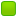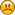###Author Topic: [SOLVED]Transformation problem  (Read 5317 times)

####elhoce

• byte
•• Posts: 16##### [SOLVED]Transformation problem
« on: April 02, 2012, 03:21:48 pm »
I try to make animations with the vertex shader using the transformation matrices calculated by bones.
Unfortunately I get enough strange animations.

I guess it comes from the fact that your calculation result is stored in the matrices JPCT.
But OpenGL expects to have an OpenGL matrix.
Are the two types of matrices compatible? Otherwise How can I convert the jpct matricx to openGl matrix without going through the method transforGL() of the JPCT class Matrix ?
To tell you the context, the treatment that you do in applySkeletonPose (animate3d class) I did it for my part in the VertexShader.

If I understand your algorithm, you apply the transformations on the mesh which is the previous animation, unlike me where the mesh is still bindPose. What should i do on the palette array to obtain the changes since the bind pose.
« Last Edit: April 03, 2012, 07:40:07 pm by elhoce »

####raft

• Moderator
••• Posts: 1993##### Re: Transformation problem
« Reply #1 on: April 02, 2012, 04:56:19 pm »
yes, AFAIK jPCT and OpenGL matrices are not compatible, that's why the method Matrix.transformToGL() exists. why can't you use that method? if you really can't, Egon can give more detail about how to convert one to other

animation calculations do not depend on previous state or animation, however the thing you need to remember is each joint's (bone) transformation is in parent joint's space (relative to its parent)

####elhoce

• byte
•• Posts: 16##### Re: Transformation problem
« Reply #2 on: April 02, 2012, 07:50:30 pm »
Thanks for your quick replyThe problem about the transformToGL call is the matrix identity (jpct) converted doesn't give me an identity matrix (OpenGL)

Thanks

####raft

• Moderator
••• Posts: 1993##### Re: Transformation problem
« Reply #3 on: April 02, 2012, 08:07:59 pm »
You're welcomeI'm not sure about Matrix thing. Egon may clarify it.

About parent relation, correct, that calculation is not done in applySkeletonPose, indeed it is done nowhere, it is exported that way by the exporter. But you have to take care of that if you programatically do animation like in ProceduralAnimationSample. Just a bit of information not sure if it will help in your case

####EgonOlsen

••• Posts: 12135##### Re: Transformation problem
« Reply #4 on: April 02, 2012, 09:16:22 pm »
transformToGL transforms your matrix in a way that vertex coordinates from jPCT will be transformed into GL's coordinate system when applying the matrix. That method's name might be a bit misleading. What this means it that an indentity matrix from jPCT wont result in the same matrix when using this method but in a matrix that will transform any jPCT coordinate into a gl coordinate as if you would have applied the identity matrix to some gl coordinate. For reference, this is what the method does:

Code: [Select]
`public void transformToGL() { mat0 *= -1f; mat0 *= -1f; mat1 *= -1f; mat1 *= -1f; mat2 *= -1f; mat2 *= -1f; mat3 *= -1f; mat3 *= -1f; }`

####elhoce

• byte
•• Posts: 16##### Re: Transformation problem
« Reply #5 on: April 02, 2012, 09:46:30 pm »
Thanks to you Egon for this precision.

Raft, my goal is to play an animation coming from an ogre xml, not to do animation by coding. So could you please tell if this bunch of code does the same thing that applySkeletonPose method :

vec4 vPos = bones[j1] * vec4(position, 1.0) * w1 + bones[j2] * vec4(position, 1.0) * w2 + bones[j3] * vec4(position, 1.0) * w3 + bones[j4] * vec4(position, 1.0) * w4

with position is the position of the current vertex
j1,j2,j3,j4 the 4 indices of joints and w1,w2,w3,w4 the four weigths associated to the current vertex.

this code is executed for every vertex (vertex shader) of the mesh that I try to animate.

Thanks for your help####raft

• Moderator
••• Posts: 1993##### Re: Transformation problem
« Reply #6 on: April 02, 2012, 10:07:17 pm »
assuming bones[jx]'s are palette matrices, yes, seems ok to me.

####elhoce

• byte
•• Posts: 16##### Re: Transformation problem
« Reply #7 on: April 03, 2012, 12:34:52 am »
Yes it's the palette array !

Now I'm trying to figure out how to interpret a matrix palette.
I have a simple animation :

<keyframes>
<keyframe time="0">
<translate x="0" y="0" z="0" />
<rotate angle="0.0">
<axis x="0" y="1" z="0" />
</rotate>
<scale x="1" y="1" z="1" />
</keyframe>
<keyframe time="10">
<translate x="0" y="0" z="0" />
<rotate angle="-30.0">
<axis x="1" y="0" z="0" />
</rotate>
<scale x="1" y="1" z="1" />
</keyframe>
<keyframe time="20">
<translate x="0" y="0" z="0" />
<rotate angle="30.0">
<axis x="1" y="0" z="0" />
</rotate>
<scale x="1" y="1" z="1" />
</keyframe>
</keyframes>
</track>

When I debuging my project, the palette matrix of the bone "Head" at "n" time is

translation : 7.8505083E-16, -0.77856064, -6.410426
rotation X axis : 1.0, -2.2415054E-17, 2.068825E-18
rotation Y axis : 2.2415054E-17, 0.9831068, -0.1830328
rotation Z axis : 2.068825E-18, 0.1830328, 0.9831068

I find the translation is wrong or this values are not about the translation ?
The result of the animation is that the head does translation animation over the z axis and  y axis; i think the rotation is interpreted as a translationI think my problem comes the interpretation of the matrix.
Could you please to help me to understand the values of the matrix in order to convert them for my vertex shader.

Thanks

####raft

• Moderator
••• Posts: 1993##### Re: Transformation problem
« Reply #8 on: April 03, 2012, 10:27:59 am »
palette matrices contain the final value that can be directly applied to vertices. that takes into account that each joint's transform is in parent's space. have a look at SkeletonPose.updateTransforms() to see how pallette matrices are calculated.

therefore, dont expect to see with eye the real transform of a vertice in palette matrix, unless relevant joint has no parent.

at first try to avoid rotations, they are much harder to imagine. you can manually edit/create a skeleton file to see the result.

reading final part of this document may also help:
http://www.okino.com/conv/skinning.htm

####elhoce

• byte
•• Posts: 16##### Re: Transformation problem
« Reply #9 on: April 03, 2012, 07:39:47 pm »
Thank you for your help, I solved my problems, my app works perfectly.####raft

• Moderator
••cool, I'm glad to hear thatso what you did is a software-hardware mixed skinning. how is the performance compared to sw only skinning? maybe you can publish what you did?